# CommonJS的模块规范

CommonJS对模块的定义十分简单，主要分为模块引用、模块定义和模块标识。

1、模块引用

`var math = require('math');//这个方法接受模块标识，以此引入一个模块的API到当前上下文中。`

2、模块定义

```//math.js
var sum = 0,
i = 0,
args = arguments,
l = args.length;
while (i < l){
sum += args[i++];
}
return sum;
};
//program.js
var math = require('math');//require()返回的对象实际上是exports
exports.increment = function(val){
}```

3、模块标识

CommonJS构建的模块导出和导入机制使得用户完全不必考虑变量污染。

##### 热门推荐
•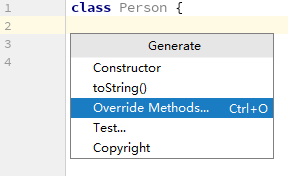浏览(612)
•浏览(391)
•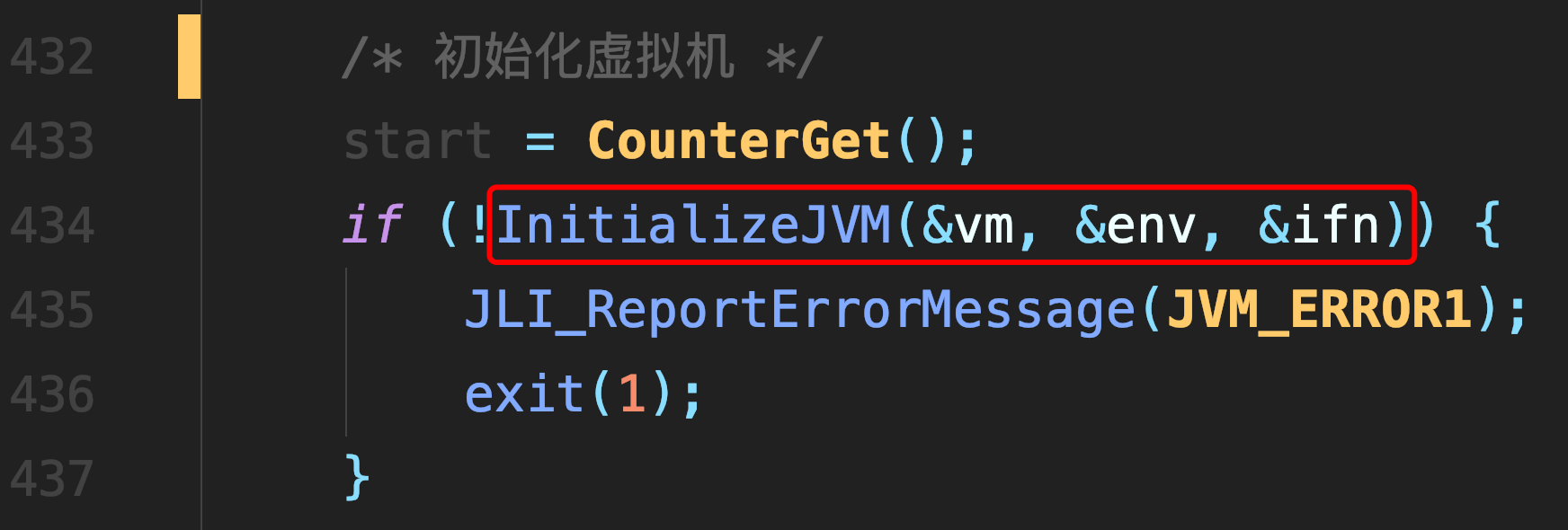浏览(365)
•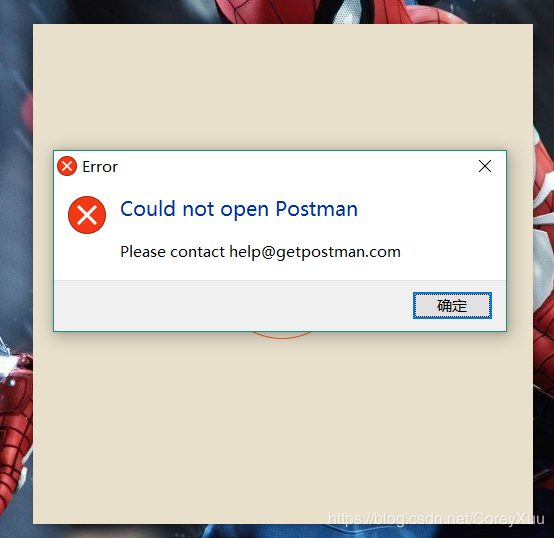浏览(351)
•浏览(347)
•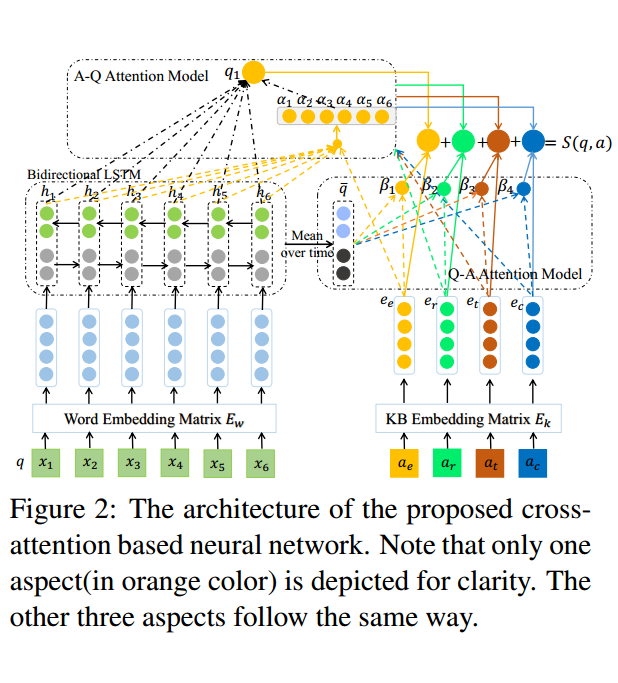浏览(310)
•浏览(292)
•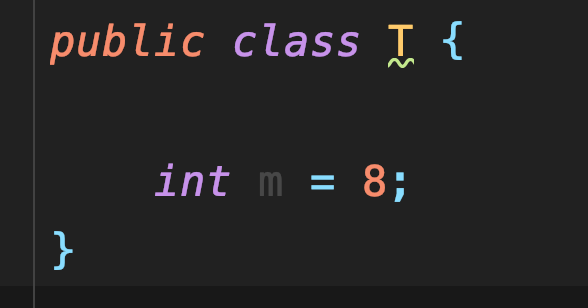浏览(281)
•浏览(267)
•浏览(251)